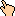## 1996-12 Solution

There are two ways to solve this, the standard way and the way requiring you to have some small understanding of number theory. First the standard way. Let's rewrite it as an addition problem first:

```  119?
+ BDCA
------
ABCD
```

The first equation derives from the ones digit: ? + A = D mod 10. But the tens digit shows that 9 + C = C, so there MUST have been a carry in the ones digit, and we know that there will be a carry in the hundreds digit, regardless of what C is (If C = 0, 1 (carry) + 9 + 0 = 10, and if C = 9, 1 (carry) + 9 + 9 = 19, so that's why there's a carry of 1 coming to Mr. Hundreds digit). So really the ones equation (which we'll call equation a) reads:

• (a) ? + A = D + 10

The hundreds digits reveal a second equation, 2 + D = B mod 10 (remember we had the carry coming from Mr. Tens). We don't know if a carry is involved from the hundreds to the thousands, but let's assume there is. This means two equations are generated (we name these assumptions b-ass and c-ass for 'assumption'):

• (b-ass) 1 (carry) + 1 + D = B + 10
• (c-ass) 1 (carry) + 1 + B = A = 2 + B

Now let's see if this is possible. Equation b-ass tells us that D must be either 8 or 9 (If it weren't, the left side of equation b-ass would never be bigger than 10, and we know the right side of b-ass is at least 10), but we know D can't be 9 because of equation a. Two single digits can't add to 19. So D must be 8, which means from equation a that ? and A must be both 9. We're done, you say, since we found out what '?' was, right? Well, let's just see about that. From equation c-ass we then know that B is 7, But then b-ass reads: 2 + 8 = 7 + 10, which is wrong. So our assumptions b-ass and c-ass were wrong. Then there is no carry from Mr. Hundreds to Mr. Thousands after all and those equations become:

• (b) 1 (carry) + 1 + D = B = 2 + D (or, rewritten) D = B - 2
• (c) 1 + B = A

Now, let's replace the variables D and A in a with their equivalences from b and c. So a rewritten becomes:

• ? = D - A + 10 = (B - 2) - (B + 1) + 10 = 10 - 3 = 7

Thus,

? = 7.

The second way involves the following understanding:

• (d) The sum of the digits of a number modulo 9 is equal to the number itself modulo 9.
• (e) If A + B = C, then ((A mod 9) + (B mod 9)) mod 9) = (C mod 9)
• (f) If A - B = C, then ((A mod 9) - (B mod 9)) mod 9) = (C mod 9)

Using d, we see that

(119? mod 9) = ((1 + 1 + 9 + ?) mod 9).

We also see from f that

((ABCD mod 9) - (BDCA mod 9)) mod 9) = (119? mod 9).

But from d again we know that

(ABCD mod 9) = ((A + B + C + D) mod 9),

and

(BDCA mod 9) = ((B + D + C + A) mod 9).

Since addition is commutative,

((A + B + C + D) mod 9) = ((B + D + C + A) mod 9)

and thus

(ABCD mod 9) = (BDCA mod 9)

and thus

((ABCD mod 9) - (BDCA mod 9)) mod 9) = (0 mod 9) = 0

and

0 = (119? mod 9) = ((1 + 1 + 9 + ?) mod 9).

So

(11 + ?) mod 9 = 0.

Thus,

? = 7.

Epilogue from Alex Berg:

This works in lots of problems, but one has to be careful because 9 is congruent to 0 modulo 9 so you would not know which to pick in case you had a choice.

WWW Maven: Dan Garcia (ddgarcia@cs.berkeley.edu)Send me feedback